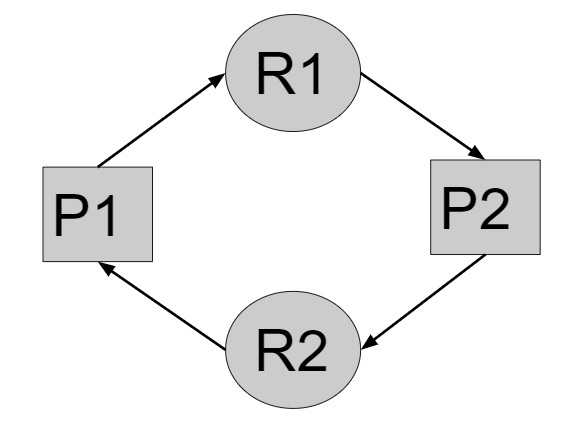# C++ Program for Deadlock free condition in Operating Systems

Given with the P number of processes in the memory and N number of needed resources by them to complete their execution and the task is to find the minimum number of resources R which should be allotted to the processes such that deadlock will never occur.

Deadlock is situation in an operating system where multiple processes residing in the memory doesn’t able to perform their execution because the resources which are needed for program execution is being hold by another resource who is waiting for some other resource for completion.

Let’s say there are two processes P1 and P2 in memory where P1 requires resource R1 and P2 requires resource R2, but the deadlock will arise when P1 hold the resource R2 and wait for the resource R1 similarly P2 hold resource R1 and wait for the resource R2.This is the example of circular wait which is one of the causes of deadlock. So, to prevent deadlock we need to calculate the number of resources which should be available for the processes such that deadlock will not occur.

R >= P * (N - 1) + 1

where, R is the Resources, P is the processes and N is the need of processes

## Example

Input-: processes = 5, need = 3
Output-: minimum required resources are: 11
Input-: Processes = 7, need = 2
Output-: minimum required resources are: 8

Approach used in the below program is as follows

• Input the number of processes and need of the processes in the memory
• Apply the formula given to calculate the number of resources required
• Display the result

## Algorithm

START
Step 1-> declare function to calculate the minimum number of resources needed
int min_resource(int process, int need)
declare int calculate = 0
set calculate = process * (need - 1) + 1
return calculate
Step 2-> In main()
Declare int process = 5 and need = 3
Call min_resource(process, need)
STOP

## Example

#include <bits/stdc++.h>
using namespace std;
//calculate minimum number of resources needed
int min_resource(int process, int need) {
int calculate = 0;
calculate = process * (need - 1) + 1;
return calculate;
}
int main() {
int process = 5, need = 3;
cout << "minimum required resources are : " <<min_resource(process, need);
return 0;
}

## Output

minimum required resources are : 11